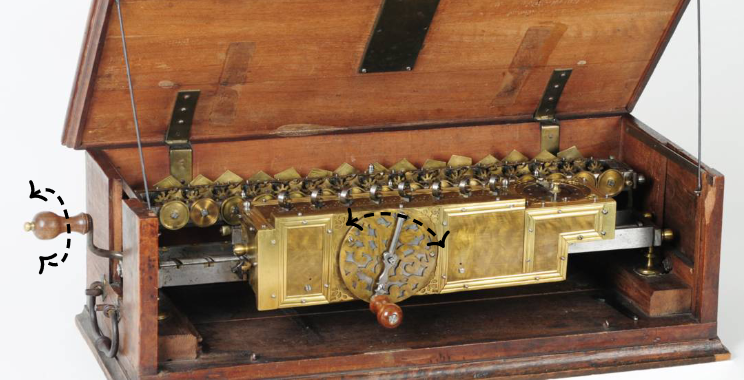# nLab reversible computation

Contents

### Context

#### Computing

intuitionistic mathematics

# Contents

## Idea

In as far as a computation is a function from input data to output result, a reversible computation is an invertible function, where the input may be recovered from knowledge of the output. More generally, as far as a computation is any morphism (under the computational trilogy), a reversible computation is an isomorphism.

Typical operations of a classical computer are not reversible: For example standard logical gates such as AND, XOR etc. are functions of the form $\{0,1\}^2 \longrightarrow \{0,1\}$ and as such not invertible. Standard classical gates which are invertible include the NOT-gate $\{0,1\} \xrightarrow{\sim} \{0,1\}$ (which switches its single input bit) and the CNOT-gate $\{0,1\}^2 \xrightarrow{\sim} \{0,1\}^2$ (which keeps the first “control” bit and switches the second bit if the first equals 1).Using just these reversible NOT- and CNOT-gates one may for instance implement the operation of incremental addition $(-)+n \;\colon\; \mathbb{Z} \xrightarrow{\sim} \mathbb{Z}$ by a fixed natural number $n$ (e.g. Kaye 2004). As opposed to binary addition $(-) + (-) \,\colon\, \mathbb{Z}^2 \xrightarrow{\;} \mathbb{Z}$, this operation is invertible, the inverse being the corresponding incremental subtraction $(-)-n \;\colon\; \mathbb{Z} \xrightarrow{\sim} \mathbb{Z}$.

This state of affairs was reflected, for instance, in Gottfried Leibniz‘s stepped reckoner machine, which performs incremental addition when its crank is turned in one direction, and the inverse incremental subtraction when the crank is turned back in the opposite direction.

But the NOT- and CNOT-gates by themselves are not universal. On the other hand, the CCNOT gate $\{0,1\}^3 \xrightarrow{\sim} \{0,1\}^3$ (which regards the first two inputs as control bits and switches the third input if these two are both 1) is both invertible as well as universal (e.g. Nielsen & Chuang 2000, §1.4.1), in that any function of the form $\{0,1\}^{n_1} \xrightarrow{\;} \{0,1\}^{n_2}$ is equal to a circuit of CCNOT gates pre-composed with some cartesian power of $\{0\} \xhookrightarrow{\;} \{0,1\}$ (introducing auxiliary “bath” or “ancilla” bits) and post-composed with some cartesian power of $\{0,1\} \to \ast$ (deleting auxiliary bits). In other words: Every irreversible classical computation on some computing system may be understood as a reversible computation on the system coupled to an ambient “bath” together with a loss of information of computation output into this bath.

Indeed, in terms of physical implementation of computation, the distinction between reversible and irreversible computation is essentially that of reversible or irreversible dynamical processes as known from thermodynamics: Fundamentally and under idealized conditions, physical processes are invertible, but “at finite temperature” there is entropy-increase associated with most processes, making them irreversible. This relation between (non-)reversible computation and thermodynamics is known as Landauer’s principle (due to Landauer 1961, review e.g. in Nielsen & Chuang 2000, §3.2.5).

While the notion of reversible computation was originally conceived in the context of classical computation, more recently its key example is understood to be quantum computation: Here the logical gates (excluding the final quantum measurement which collapses the quantum state) are linear functions which are unitary operator and hence necessarily invertible (see Frank 2003 for emphasis).

Therefore, the practical problem of implementing quantum computation faces analogous thermodynamical issues as embodied by Landauer’s principle, which are the reason for the need of quantum error correction and/or topological quantum stabilization.

Original articles:

Review:

Review of current developments (as of 2020):

• B. Aman et al., Foundations of Reversible Computation, in: Reversible Computation: Extending Horizons of Computing. RC 2020 Lecture Notes in Computer Science 12070, Springer (2020) [doi:10.1007/978-3-030-47361-7_1]